Large Table Example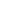This example shows how to draw a multi-page table. ABCpdf does not provide table drawing routines itself so this example uses a Table Class to position the table elements. You can find the full project and classes under the ABCpdf menu item. The project includes code for laying out a small table, a large table spreading over more than one page, an invoice and a product list.Setup

 We start by creating our document object and reading the data for our table. For the purposes of this example we'll assume that our data is in a standard tab delimited format.   ```string theText = File.ReadAllText(Server.MapPath("text7.txt")); using (Doc doc = new Doc()) {   // set up document   doc.FontSize = 12;   doc.Rect.Inset(20, 20); ``` ```Dim theText As String = File.ReadAllText(Server.MapPath("text7.txt")) Using doc As New Doc()   ' set up document   doc.FontSize = 12   doc.Rect.Inset(20, 20) ```Rotate

 We create a new table object passing in values to tell it what rectangle it can occupy (it takes the current document rectangle) and how many columns of data it should be prepared for. Columns are assigned relative widths and expand horizontally to fit the table rectangle. Here we're specifying six columns and a number of relative widths. We're padding the cells so there are gaps between the rows and columns. Finally we specify a header which repeats as new pages are added.   ```  PDFTable theTable = new PDFTable(doc, 6);   // some columns extra width   theTable.SetColumnWidths(new double[] { 2, 1, 3, 2, 1, 4 });   theTable.CellPadding = 5;   theTable.RepeatHeader = true; ``` ```  Dim theTable As New PDFTable(doc, 6)   ' some columns extra width   theTable.SetColumnWidths(New Double() {2, 1, 3, 2, 1, 4})   theTable.CellPadding = 5   theTable.RepeatHeader = True ```We iterate through the table data adding rows and columns as we go. Every time we add a row we check to see if the page number has changed and restart the shading if it has. This ensures the header is always unshaded. Finally we save the document.   ```  theText = theText.Replace("\r\n", "\r");   string[] theRows = theText.Split(new char[] { '\r' });   int thePage = 1;   bool theShade = false;   for (int i = 0; i < theRows.Length; i++) {     theTable.NextRow();     string[] theCols = theRows[i].Split(new char[] { '\t' });     theTable.AddTextStyled(theCols);     if (doc.PageNumber > thePage) {       thePage = doc.PageNumber;       theShade = true;     }     if (theShade)       theTable.FillRow("200 200 200", theTable.Row);     theShade = !theShade;   }   doc.Flatten();   doc.Save(Server.MapPath("table2.pdf")); } ``` ```  theText = theText.Replace(vbCr & vbLf, vbCr)   Dim theRows As String() = theText.Split(New Char() {ControlChars.Cr})   Dim thePage As Integer = 1   Dim theShade As Boolean = False   Dim i As Integer = 0   While i < theRows.Length     theTable.NextRow()     Dim theCols As String() = theRows(i).Split(New Char() {ControlChars.Tab})     theTable.AddTextStyled(theCols)     If doc.PageNumber > thePage Then       thePage = doc.PageNumber       theShade = True     End If     If theShade Then       theTable.FillRow("200 200 200", theTable.Row)     End If     theShade = Not theShade     System.Math.Max(System.Threading.Interlocked.Increment(i),i - 1)   End While   doc.Flatten()   doc.Save(Server.MapPath("table2.pdf")) End Using ```Results

Using a large quantity of input data. We get the following output.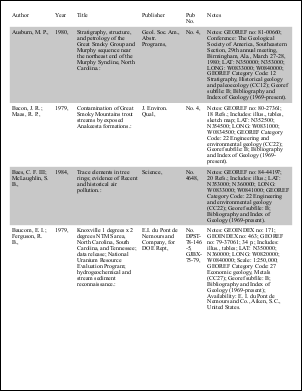table2.pdf - [Page 1]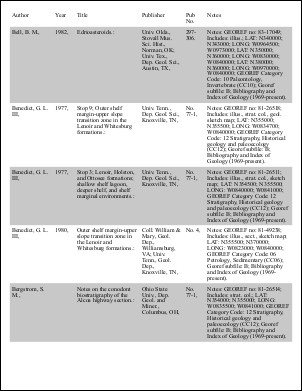table2.pdf - [Page 2]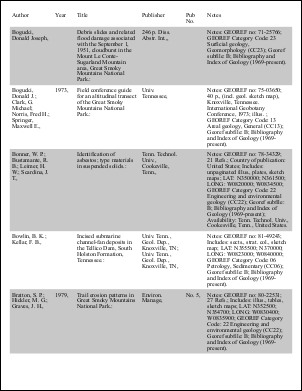table2.pdf - [Page 3]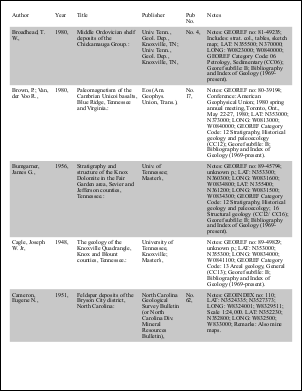table2.pdf - [Page 4]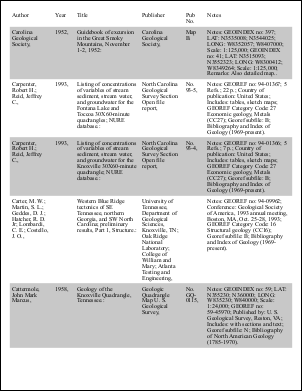table2.pdf - [Page 5]